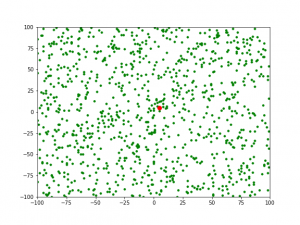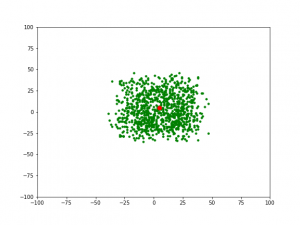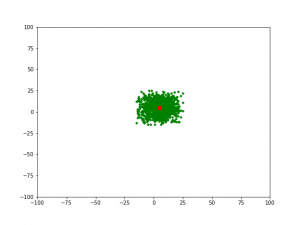# Genetic Algorithm for Reinforcement Learning : Python implementation

Most beginners in Machine Learning start with learning Supervised Learning techniques such as classification and regression. However, one of the most important paradigms in Machine Learning is Reinforcement Learning (RL) which is able to tackle many challenging tasks. It is an aspect of Machine learning where an agent learns to behave in an environment, by performing certain actions and observing the rewards(results) which it gets from those actions.

In Reinforcement Learning, we give the machines a few inputs and actions, and then, reward them based on the output. Reward maximization is the end goal. It is just like a little baby who knows nothing at first is left alone in an environment and then after a little understanding tends to know things around itself.

Here, we are just going to build an algorithm based on the genetic mutation of a population when attacked by a virus. In the first generation of our population only a few fittest ones will be able to survive, whereas as the generations will pass, the new generations will be much stronger against the virus than their ancestors. It is a basic algorithm which just gives an idea of how these things work. Anyone with the basic knowledge of python and some libraries like numpy, matplotlib, etc can easily understand this code. This is just for the introduction and to provide the surface level knowledge about Reinforcement Learning.

## Genetic Algorithm –

Libraries Used:

• numpy : we’ll be using numpy arrays and other basic calculation functionalities from this library
• matplotlib : we’ll be using matplotlib.pyplot functionality in order to plot the graphs for the visual understanding of the algorithm.

In this program, we’ll define 3 main functions in order to generate the next generation of the population which is genetically more powerful than the previous ones.

The three main functions used are:

populate: This is used to generate the population and then appending it to a list. This function accepts the attributes like the number of features in the population and the size of it.

reduction: This function is used to reduce the size of the population and allows only the 100 most fittest ones to survive. These fit ones will be the ones used to generate the next generation of the population.

cross: This function is used for the process of cross-breeding between the ones that are left in order to generate a whole new generation of the population which will be much more immune towards the virus.

Below is the implementation –

 `import` `numpy as np ` `import` `matplotlib.pyplot as plt ` ` `  `# specifying the size for each and  ` `# every matplotlib plot globally ` `plt.rcParams[``'figure.figsize'``] ``=` `[``8``, ``6``]  ` ` `  `# defining list objects with range of the graph ` `x1_range ``=` `[``-``100``, ``100``] ` `x2_range ``=` `[``-``100``, ``100``] ` ` `  `# empty list object to store the population ` `population ``=` `[] ` ` `  `# this function is used to generate the population ` `# and appending it to the population list defined above ` `# it takes the attributes as no. of features in a  ` `# population and size that we have in it ` `def` `populate(features, size ``=` `1000``): ` `     `  `    ``# here we are defining the coordinate  ` `    ``# for each entity in a population ` `    ``initial ``=` `[]  ` `     `  `    ``for` `_ ``in` `range``(size): ` `        ``entity ``=` `[] ` `        ``for` `feature ``in` `features: ` `             `  `            ``# this * feature variable unpacks a list  ` `            ``# or tuple into position arguments. ` `            ``val ``=` `np.random.randint(``*``feature) ` `            ``entity.append(val) ` `        ``initial.append(entity) ` `     `  `    ``return` `np.array(initial) ` ` `  `# defining the virus in the form of numpy array ` `virus ``=` `np.array([``5``, ``5``]) ` ` `  `# only the 100 fit ones will survive in this one ` `def` `fitness(population, virus, size ``=` `100``): ` `     `  `    ``scores ``=` `[] ` `     `  `    ``# enumerate also provides the index as for the iterator ` `    ``for` `index, entity ``in` `enumerate``(population):  ` `        ``score ``=` `np.``sum``((entity``-``virus)``*``*``2``) ` `        ``scores.append((score, index)) ` `     `  `    ``scores ``=` `sorted``(scores)[:size] ` `     `  `    ``return` `np.array(scores)[:, ``1``] ` ` `  `# this function is used to plot the graph ` `def` `draw(population, virus): ` `    ``plt.xlim((``-``100``, ``100``)) ` `    ``plt.ylim((``-``100``, ``100``)) ` `    ``plt.scatter(population[:, ``0``], population[:, ``1``], c ``=``'green'``, s ``=` `12``) ` `    ``plt.scatter(virus[``0``], virus[``1``], c ``=``'red'``, s ``=` `60``)  ` `     `  `     `  `def` `reduction(population, virus, size ``=` `100``): ` `     `  `    ``# only the index of the fittest ones ` `    ``# is returned in sorted format ` `    ``fittest ``=` `fitness(population, virus, size)  ` ` `  `    ``new_pop ``=` `[] ` `     `  `    ``for` `item ``in` `fittest: ` `        ``new_pop.append(population[item]) ` `         `  `    ``return` `np.array(new_pop) ` ` `  `# cross mutation in order to generate the next generation ` `# of the population which will be more immune to virus than previous ` `def` `cross(population, size ``=` `1000``): ` `     `  `    ``new_pop ``=` `[] ` `     `  `    ``for` `_ ``in` `range``(size): ` `        ``p ``=` `population[np.random.randint(``0``, ``len``(population))] ` `        ``m ``=` `population[np.random.randint(``0``, ``len``(population))] ` `     `  `        ``# we are only considering half of each  ` `        ``# without considering random selection ` `        ``entity ``=` `[] ` `        ``entity.append(``*``p[:``len``(p)``/``/``2``]) ` `        ``entity.append(``*``m[``len``(m)``/``/``2``:]) ` `         `  `        ``new_pop.append(entity) ` `     `  `    ``return` `np.array(new_pop) ` ` `  `# generating and adding the random features to ` `# the entity so that it looks more distributed ` `def` `mutate(population): ` `     `  `    ``return` `population ``+` `np.random.randint(``-``10``, ``10``, ``2000``).reshape(``1000``, ``2``) ` ` `  ` `  `# the complete cycle of the above steps ` `population ``=` `populate([x1_range, x2_range], ``1000``) ` ` `  `# gens is the number of generation ` `def` `cycle(population, virus, gens ``=` `1``):  ` `     `  `    ``# if we change the value of gens, we'll get  ` `    ``# next and genetically more powerful generation ` `    ``# of the population ` `    ``for` `_ ``in` `range``(gens): ` `        ``population ``=` `reduction(population, virus, ``100``) ` `        ``population ``=` `cross(population, ``1000``) ` `        ``population ``=` `mutate(population) ` `         `  `    ``return` `population ` ` `  `population ``=` `cycle(population, virus) ` ` `  `draw(population, virus) `

Output:

1) For generation 1, when gens=02) For generation 2, when gens=13) For generation 3, when gens=2My Personal Notes arrow_drop_upCheck out this Author's contributed articles.

If you like GeeksforGeeks and would like to contribute, you can also write an article using contribute.geeksforgeeks.org or mail your article to contribute@geeksforgeeks.org. See your article appearing on the GeeksforGeeks main page and help other Geeks.

Please Improve this article if you find anything incorrect by clicking on the "Improve Article" button below.

Article Tags :
Practice Tags :

Be the First to upvote.

Please write to us at contribute@geeksforgeeks.org to report any issue with the above content.